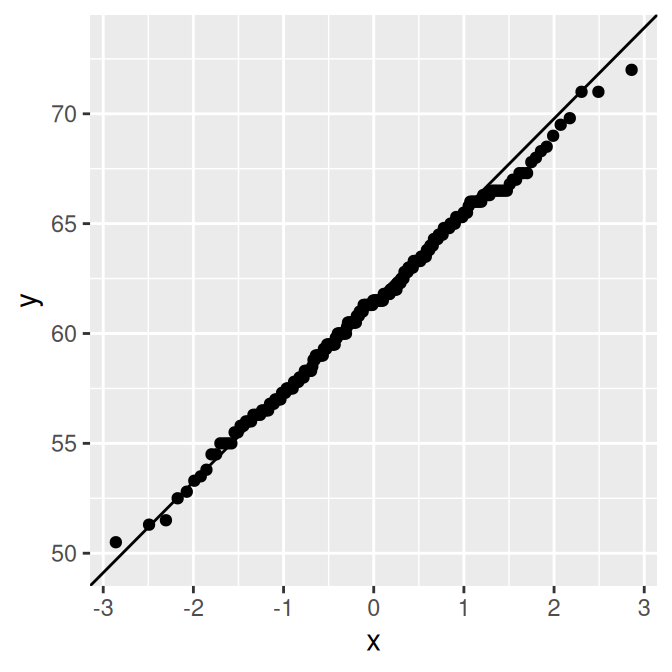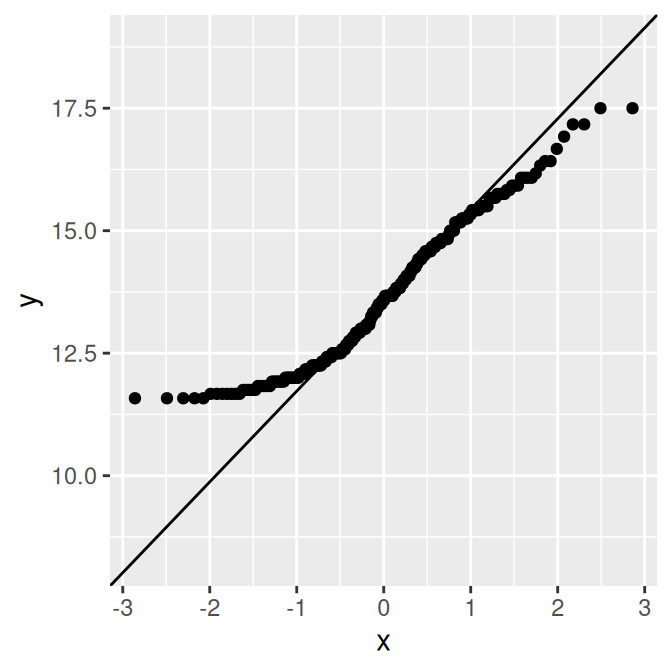## 13.13 Creating a QQ Plot

### 13.13.1 Problem

You want to make a quantile-quantile (QQ) plot to compare an empirical distribution to a theoretical distribution.

### 13.13.2 Solution

Use `geom_qq()` and `geom_qq_line()` to compare to a normal distribution (Figure 13.25):

``````library(gcookbook) # For the data set

ggplot(heightweight, aes(sample = heightIn)) +
geom_qq() +
geom_qq_line()

ggplot(heightweight, aes(sample = ageYear)) +
geom_qq() +
geom_qq_line()``````Figure 13.25: QQ plot of height, which is close to normally distributed (left); QQ plot of age, which is not normally distributed (right)

### 13.13.3 Discussion

The points for `heightIn` are close to the line, which means that the distribution is close to normal. In contrast, the points for `ageYear` veer far away from the line, especially on the left, indicating that the distribution is skewed. A histogram may also be useful for exploring how the data is distributed.

See `?stat_qq` for information on comparing data to theoretical distributions other than the normal distribution.